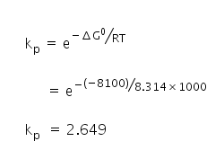# For the water gas reaction c(g)+h2o(g)=co(g)+h2(g )

For the water gas reaction c(g)+h2o(g)=co(g)+h2(g ) the standard Gibbs free energy for the reaction at 1000k is -8.1 k3 /mol. Calculate its Equlipriam constant. (R=8.314×10-3k3/k/mol).

Given:
Gibbs free energy ΔG0 = −8.1 kJ
= 8100 J
Temperature, T = 1000 K
Gas constant, R = 8.314 J/mol K
We know,
Equilibrium constant kp =